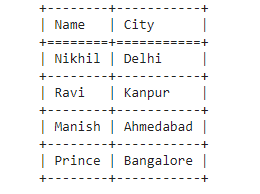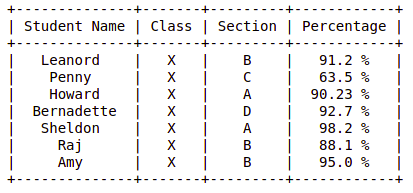# How to make a table in Python?

• Difficulty Level : Easy
• Last Updated : 22 Feb, 2022

In this article, we are going to discuss how to make a table in Python. Python provides vast support for libraries that can be used for creating different purposes. In this article we will talk about two such modules that can be used to create tables.

Method 1: Using Tabulate module

The tabulate() method is a method present in the tabulate module which creates a text-based table output inside the python program using any given inputs. It can be installed using the below command

`pip install tabulate`

Below are some examples which depict how to create tables in python:

Example 1

## Python3

 `# import module` `from` `tabulate ``import` `tabulate`   `# assign data` `mydata ``=` `[` `    ``[``"Nikhil"``, ``"Delhi"``], ` `    ``[``"Ravi"``, ``"Kanpur"``], ` `    ``[``"Manish"``, ``"Ahmedabad"``], ` `      ``[``"Prince"``, ``"Bangalore"``]` `]`   `# create header` `head ``=` `[``"Name"``, ``"City"``]`   `# display table` `print``(tabulate(mydata, headers``=``head, tablefmt``=``"grid"``))`

Output:Example 2

## Python3

 `# import module` `from` `tabulate ``import` `tabulate`   `# assign data` `mydata ``=` `[` `    ``[``'a'``, ``'b'``, ``'c'``],` `      ``[``12``, ``34``, ``56``],` `      ``[``'Geeks'``, ``'for'``, ``'geeks!'``]` `]`   `# display table` `print``(tabulate(mydata))`

Output:Method 2: Using PrettyTable module

PrettyTable class inside the prettytable library is used to create relational tables in Python. It can be installed using the below command.

`pip install prettytable `

Example:

## Python3

 `from` `prettytable ``import` `PrettyTable `   `# Specify the Column Names while initializing the Table ` `myTable ``=` `PrettyTable([``"Student Name"``, ``"Class"``, ``"Section"``, ``"Percentage"``]) `   `# Add rows ` `myTable.add_row([``"Leanord"``, ``"X"``, ``"B"``, ``"91.2 %"``]) ` `myTable.add_row([``"Penny"``, ``"X"``, ``"C"``, ``"63.5 %"``]) ` `myTable.add_row([``"Howard"``, ``"X"``, ``"A"``, ``"90.23 %"``]) ` `myTable.add_row([``"Bernadette"``, ``"X"``, ``"D"``, ``"92.7 %"``]) ` `myTable.add_row([``"Sheldon"``, ``"X"``, ``"A"``, ``"98.2 %"``]) ` `myTable.add_row([``"Raj"``, ``"X"``, ``"B"``, ``"88.1 %"``]) ` `myTable.add_row([``"Amy"``, ``"X"``, ``"B"``, ``"95.0 %"``]) `   `print``(myTable)`

Output:My Personal Notes arrow_drop_up
Recommended Articles
Page :